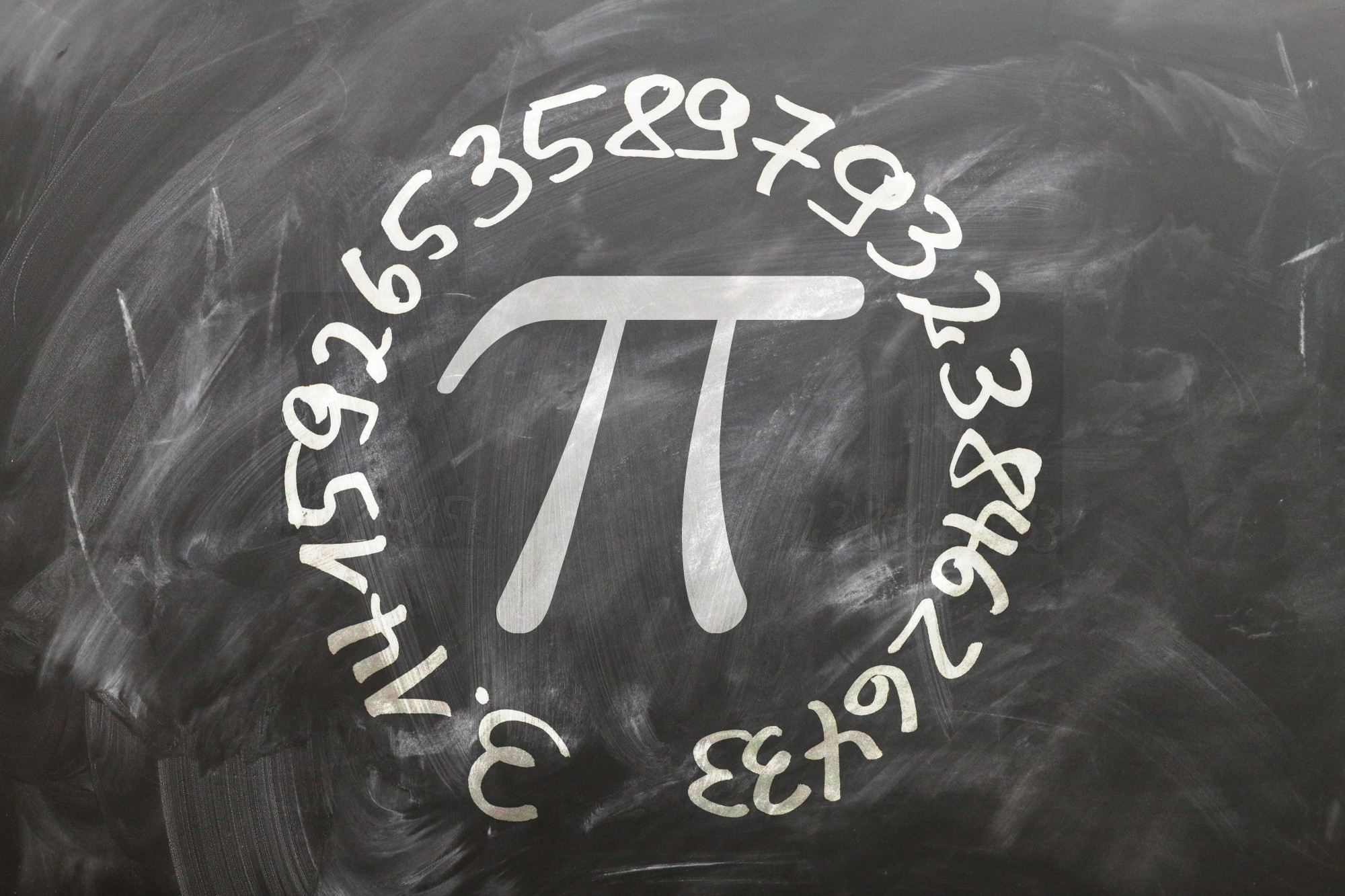/How Do You Read or Interpret an Equivalent Ratio?## How Do You Read or Interpret an Equivalent Ratio?

Back in high school, math tasks like solving equations caused no end of math anxiety for a lot of us. But often, without even realizing it, we make use of math every day.

For example, you might have thought you saw the last of ratios in math class, but learning how to read an equivalent ratio can help with everything from baking to converting foreign currencies

Not sure how to interpret a ratio? Keep reading to find out more about everyday ratio use!

## How Do I Read a Ratio?

Before we get into the equivalent ratio meaning, you need to learn how to read a ratio.

Ratios compare two numbers in relation to each other. Usually, these numbers represent some kind of measurement or quantity. For example, if you have a fruit bowl containing six oranges and two apples, the ratio of oranges to apples is 6 to 2, or 6:2.

Ratios can be dependent, as they are in a recipe, or independent, as they are in the fruit bowl example.

### Dependent Ratios

A basic pizza dough recipe might call for four cups of flour and a cup of water, meaning a ratio of 4:1. Want to make twice as much dough? You’ll have to double up, meaning eight cups of flour and two cups of water, or 8:2.

Or, for a DIY window-cleaning solution you might need to mix one part water with one part white vinegar. A ‘part’ can be any quantity, but you must stay true to the ratio by using the same unit of measurement for both ingredients.

### Independent Ratios

You can also use ratios to demonstrate the relation between two unconnected quantities. Using the fruit bowl example, all the 6:2 ratio does is compare the quantities of these two fruits. If we added another piece of fruit or ate one, we would have to change the ratio to represent the new proportional difference.

## How Can I Calculate an Equivalent Ratio?

Equivalent ratios are especially useful when you’re trying to understand both dependent and independent ratios with larger numbers. But, what is an equivalent ratio?

An equivalent ratio shows the same relationship between two numbers as the original ratio. To find an equivalent ratio, you can either divide or multiply each number in the ratio by the same number.

We actually used an equivalent ratio in the pizza dough recipe when we multiplied both ingredients by two. Here, the equivalent ratios 4:1 and 8:2 represent the same relationship of four times more flour than water.

Although, when you’re dealing with large numbers, you can see more here about how to use an equivalent ratio calculator to guarantee accuracy and make the task easier.

## Learning How to Read an Equivalent Ratio

There’s a good chance that you might never have heard of an equivalent ratio before today. But now we’ve explained the equivalent ratio meaning, we’re betting that everything is a lot clearer.

And, if you’ve ever multiplied or divided ingredient quantities in a recipe, you already knew how to calculate equivalent ratios, you just didn’t know it!

For more great learning tips and all the latest tutorials, be sure to check out our other blog posts.